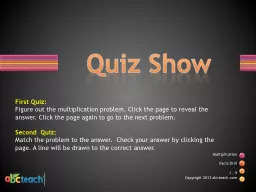# Quiz ShowEmbed code:

## Quiz Show

Download Presentation - The PPT/PDF document "Quiz Show" is the property of its rightful owner. Permission is granted to download and print the materials on this web site for personal, non-commercial use only, and to display it on your personal computer provided you do not modify the materials and that you retain all copyright notices contained in the materials. By downloading content from our website, you accept the terms of this agreement.

### Presentations text content in Quiz Show

Slide1

Quiz Show

Multiplication

Facts Drill

First Quiz:Figure out the multiplication problem. Click the page to reveal the answer. Click the page again to go to the next problem. Second Quiz: Match the problem to the answer. Check your answer by clicking the page. A line will be drawn to the correct answer.

Slide2

What is the answer to 2 x 3 ?

6

Did you know?

2 + 2 + 2 = 2 x 3

Slide3

What is the answer to 1 x 2 ?

2

Did you know?

1 + 1 = 1 x 2

Slide4

What is the answer to 1 x 3 ?

3

Did you know?

1 x 3 = 3 x 1

Slide5

What is the answer to 3 x 3 ?

9

Did you know?

You can count by

3

Slide6

What is the answer to 2 x 2 ?

4

Did you know?

2 + 2 = 2 + 2

Slide7

What is the answer to 2 x 2 ?

4

Did you know?

2 + 2 = 2 + 2

Slide8

What is the answer to 4 x 3 ?

12

Slide9

What is the answer to 2 x 5 ?

10

Slide10

What is the answer to 3 x 9 ?

27

Slide11

What is the answer to 5 x 5 ?

25

Slide12

What is the answer to 7 x 8 ?

56

Slide13

What is the answer to 6 x 4 ?

24

Slide14

What is the answer to 3 x _ = 12

4

Did you know?

You can add to find your

answer by circling groups of 3

and then counting the groups.

Slide15

What is the answer to 3 x _ = 18

6

Did you know?

You can add to find your

answer by circling groups of 3

and then counting the groups.

Slide16

What is the answer to 2 x _ = 8

4

Did you know?

You can add to find your

answer by circling groups of 2

and then counting the groups.

Slide17

What is the answer to 2 x _ = 6

2

Did you know?

You can add to find your

answer by circling groups of 3

and then counting the groups.

Slide18

5 x 5

7 x 8

2 x 10

3 x 3

4x8

32

20

25

56

9

Match the

purple

column to the

yellow

column.

Slide19

5 x 3

2 x 8

2 x 6

2 x 3

4 x 9

36

12

15

16

6

Match the

purple

column to the

yellow

column.

Slide20

4 x __ = 20

6 x __ = 36

7 x __ = 28

3 x __ = 27

2 x __ = 16

8

4

5

6

9

Match the purple column to the yellow column.Click the problem to check your answer.